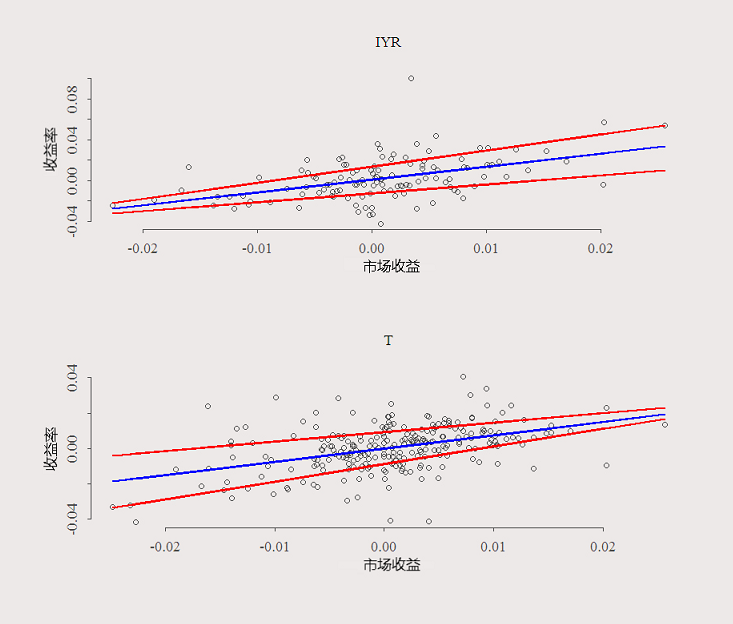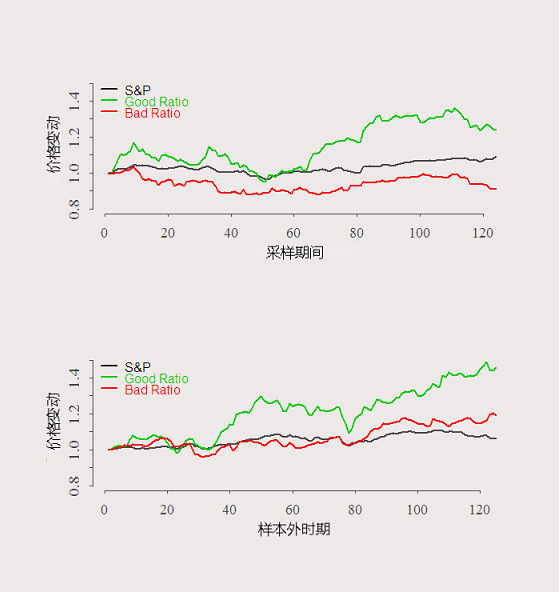# R语言分位数回归预测筛选有上升潜力的股票

## 现在，分位数回归已被确立为重要的计量经济学工具。dat0 = getSymbols(sy, src="yahoo", from=start, to=end,
auto.assign = F, warnings = FALSE,symbol.lookup = F)

#查询最近365天：
dat <- gtint(sym = c(tickers,"SPY"),365)
# 将样品划分成两部分

ins <- n/2
# 在0.2和0.8之间查找斜率

Tau = c(.2,.8)

for (j in 1:(l-1)
for (i in 1:length(Tau)
qslope[i,j] = rq(dat$ret[2:ins,j~dat$ret[2:ins,l, tau = Tau[i])$coef # 确定哪些股票有用： dat$ret <- dat$ret[,rat0<2 & rat0>(-2)] ## 画图 plot(dat$ret[1:ins,which.max(rat)]~dat$ret[1:ins,l] plot(dat$ret[1:n,which.min(rat)]~dat$ret[1:n,l], title(nam)我们使用样本的前半部分来选择我们要使用的股票。假设我们以最差的比率做空股票，并以最佳的比率做多股票。 # R语言时间序列分析模型：ARIMA-ARCH / GARCH模型分析股票价格 dat$p <- dat$p[,rat0<2 & rat0>(-2)] plot(dat$p[1:ins,l]/dat$p[1,l], ty = "l", ylim = c(.8,1.5), plot(dat$p[ins:n,l]/dat\$p[ins,l], ty = "l", ylim = c(.8,1.5), xlab = "样本外时期",)Kaizong Ye拓端研究室（TRL）的研究员。

​非常感谢您阅读本文，如需帮助请联系我们！QQ在线咨询

15121130882

0571-63341498

## 关注有关新文章的微信公众号

This will close in 0 seconds